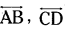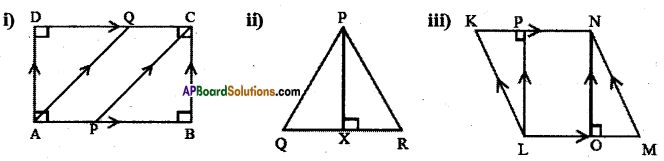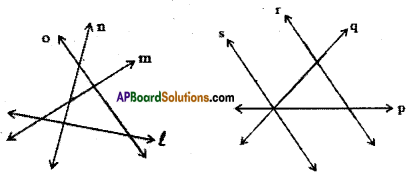# AP Board 6th Class Maths Solutions Chapter 8 Basic Geometric Concepts Ex 8.3

AP State Syllabus AP Board 6th Class Maths Solutions Chapter 8 Basic Geometric Concepts Ex 8.3 Textbook Questions and Answers.

## AP State Syllabus 6th Class Maths Solutions 8th Lesson Basic Geometric Concepts Ex 8.3Question 1.
Given ” $$\overline{\mathrm{AB}} / / \overline{\mathrm{CD}}$$, l ⊥ m”. Which are perpendicular? Which are parallel?
Solution:are parallel lines (// is the symbol for parallel),
l, m are perpendicular lines (⊥ is the symbol for perpendicular).

Question 2.
Write the set of parallels and perpendiculars in the given by using symbols.Solution:
a) $$\overline{\mathrm{AB}}, \overline{\mathrm{DC}}$$ are parallel lines, we denote this by writing $$\overline{\mathrm{AB}} / / \overline{\mathrm{DC}}$$ and can be read as $$\overline{\mathrm{AB}}$$ is parallel to $$\overline{\mathrm{DC}}$$
b) $$\overline{\mathrm{AD}}, \overline{\mathrm{BC}}$$ are parallel lines. We denote this by writing $$\overline{\mathrm{AD}} / / \overline{\mathrm{BC}}$$ and can be read as $$\overline{\mathrm{AD}}$$ is parallel to $$\overline{\mathrm{BC}}$$
c) $$\overline{\mathrm{AQ}}, \overline{\mathrm{PC}}$$ are parallel lines. We denote this by writing $$\overline{\mathrm{AQ}} / / \overline{\mathrm{PC}}$$ and can be read as $$\overline{\mathrm{AQ}}$$ is parallel to $$\overline{\mathrm{PC}}$$.
d) $$\overline{\mathrm{AB}}, \overline{\mathrm{AD}}$$ are perpendicular lines. We denote this by writing $$\overline{\mathrm{AB}} \perp \overline{\mathrm{AD}}$$ and can be read as $$\overline{\mathrm{AB}}$$ is perpendicular to $$\overline{\mathrm{AD}}$$.
e) $$\overline{\mathrm{AB}}, \overline{\mathrm{BC}}$$ are perpendicular lines. We denote this by writing $$\overline{\mathrm{AB}} \perp \overline{\mathrm{BC}}$$ and can be read as $$\overline{\mathrm{AB}}[latex] is perpendicular to [latex]\overline{\mathrm{BC}}$$ .
f) $$\overline{\mathrm{BC}}, \overline{\mathrm{CD}}$$ are perpendicular lines, we denote this by writing $$\overline{\mathrm{BC}} \perp \overline{\mathrm{CD}}$$ and can be read as $$\overline{\mathrm{BC}}$$ is perpendicular to $$\overline{\mathrm{CD}}$$.
g) $$\overline{\mathrm{CD}}, \overline{\mathrm{AD}}$$ are perpendicular lines, we denote this by writing $$\overline{\mathrm{CD}} \perp \overline{\mathrm{AD}}$$ and can be read as $$\overline{\mathrm{CD}}$$ is perpendicular to $$\overline{\mathrm{AD}}$$.

ii) $$\overline{\mathrm{PX}}, \overline{\mathrm{QR}}$$ are perpendicular lines, we denote this by writing $$\overline{\mathrm{PX}} \perp \overline{\mathrm{QR}}$$ and can be read as $$\overline{\mathrm{PX}}$$ is perpendicular to $$\overline{\mathrm{QR}}$$.

iii) a) $$\overline{\mathrm{LM}}, \overline{\mathrm{KN}}$$ are parallel lines. We denote this by writing $$\overline{\mathrm{LM}} / / \overline{\mathrm{KN}}$$ and can be read as $$\overline{\mathrm{LM}}$$ is parallel to $$\overline{\mathrm{KN}}$$.
b) $$\overline{\mathrm{MN}}, \overline{\mathrm{LK}}$$ are parallel lines. We denote this by writing $$\overline{\mathrm{MN}} / / \overline{\mathrm{LK}}$$ and can be read as $$\overline{\mathrm{MN}}$$ is parallel to $$\overline{\mathrm{LK}}$$.
c) $$\overline{\mathrm{ON}}, \overline{\mathrm{LP}}$$ are parallel lines. We denote this by writing $$\overline{\mathrm{ON}} / / \overline{\mathrm{LP}}$$ and can be read
as $$\overline{\mathrm{ON}}$$ is parallel to $$\overline{\mathrm{LP}}$$
d) $$\overline{\mathrm{LM}}, \overline{\mathrm{ON}}$$ are perpendicular lines, we denote this by writing $$\overline{\mathrm{ON}} \perp \overline{\mathrm{LM}}$$ and can be read as $$\overline{\mathrm{ON}}$$ is perpendicular to $$\overline{\mathrm{LM}}$$.
e) $$\overline{\mathrm{LP}}, \overline{\mathrm{KN}}$$ are perpendicular lines, we denote this by writing $$\overline{\mathrm{LP}} \perp \overline{\mathrm{KN}}$$ and can be read as $$\overline{\mathrm{LP}}$$ is perpendicular to $$\overline{\mathrm{KN}}$$.Question 3.
From the given figure find out the Intersecting lines and concurrent lines.Solution:
i) Intersecting lines : (l, m); (l, n); (n, o); (m, o); (l, o); (m, n)
ii) Intersecting lines: (p, q); (p, r); (p, s); (q, r); (q, s)
Concurrent lines : (p, q, s)